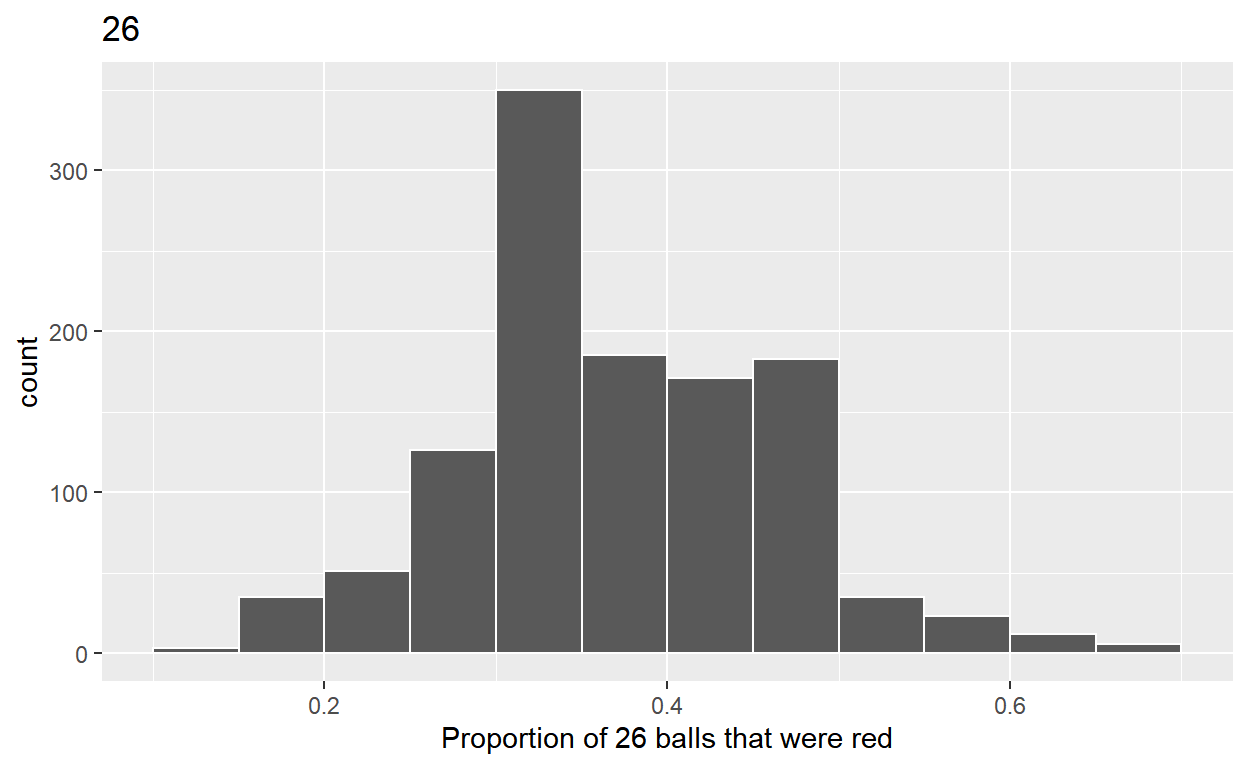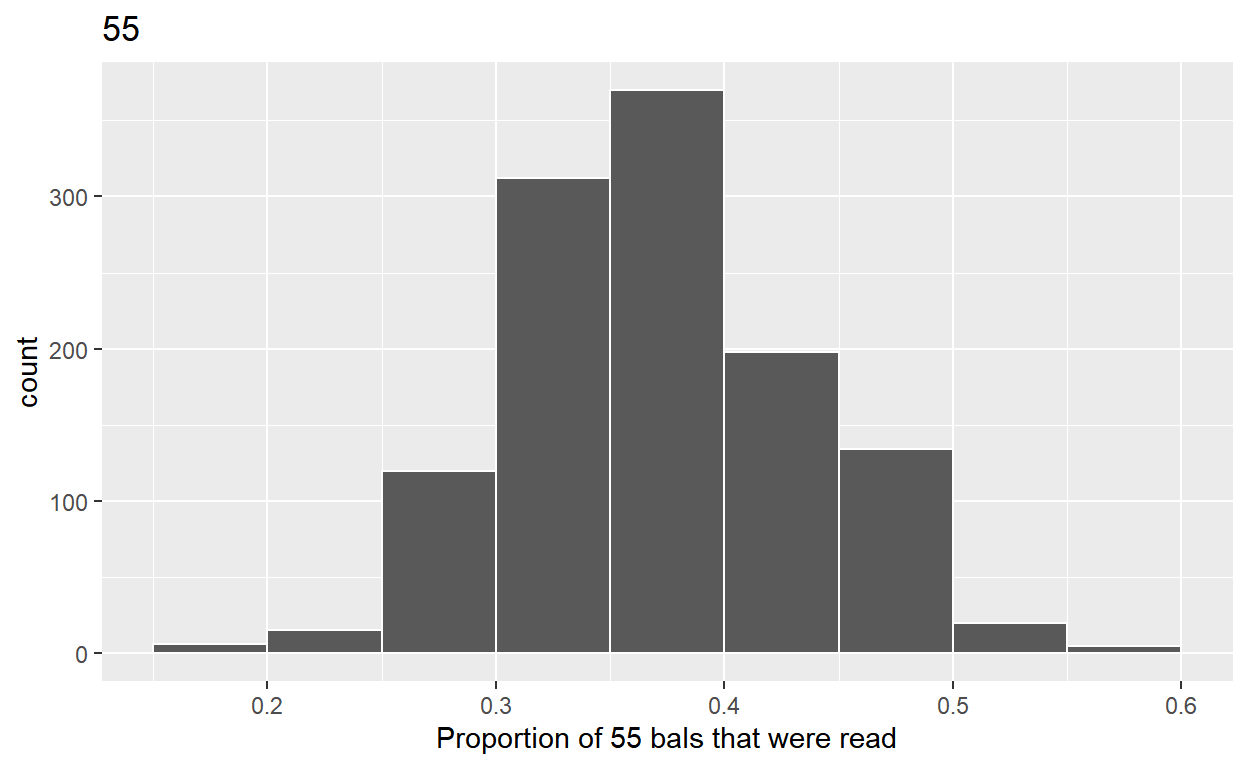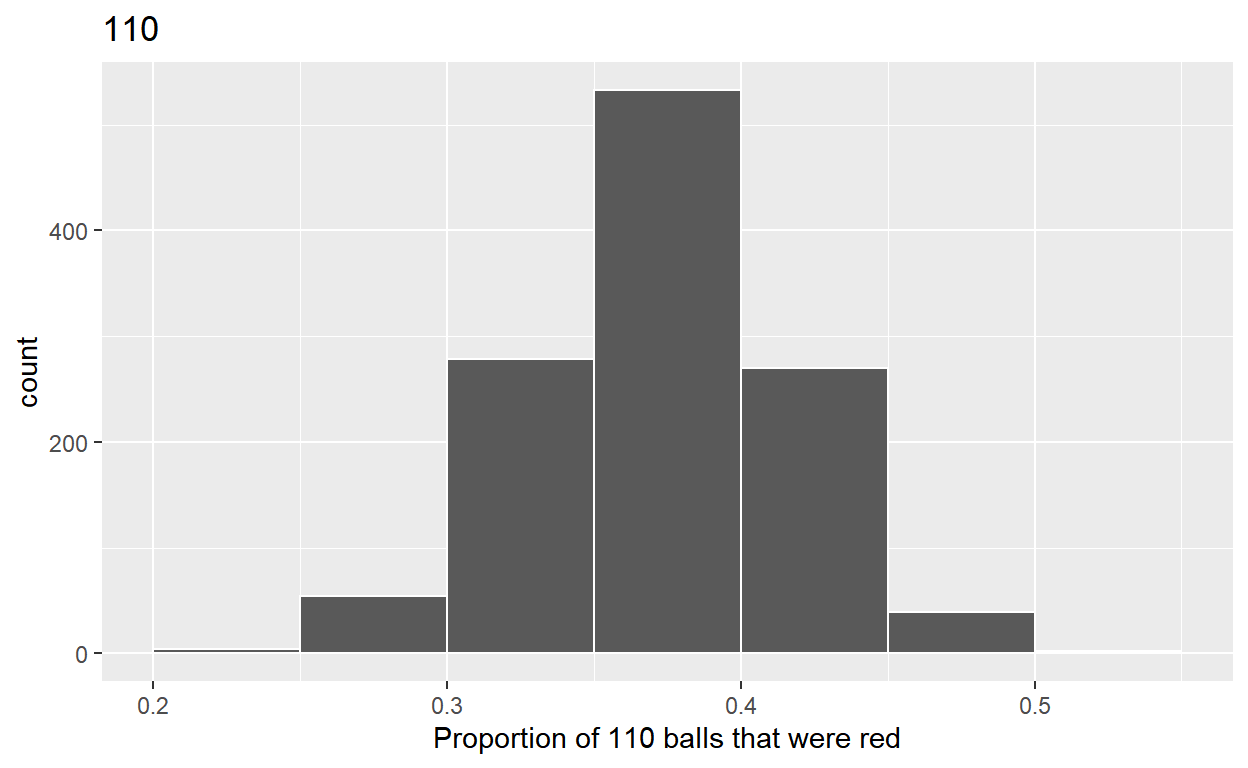# Sampling

Chapter 7 of ModernDive. Code for quiz 11.

Load the R packages we will use

``````library(tidyverse)
library(moderndive)
``````

Question: Modify the code for comparing different sample sizes from the virtual `bowl`

# Segment 1: Sample size = 26

1A.

• Take 1180 samples of size 26 instead of 1000 replicates of 25 from the `bowl` dataset.

• Assign the output to `virtual_samples_26`

``````virtual_samples_26 <- bowl %>%
rep_sample_n(size = 26, reps = 1180)
``````

1B.

• Compute the resulting 1180 replicates of proportion Red.

• Start with `virtual_samples_26` THEN

• `group_by` replicate THEN

• Create variable red equal to the sum of all the red balls

• Create variable `prop_red` equal to the variable red/26

• Assign the output to `virtual_prop_red_26`

``````virtual_prop_red_26 <- virtual_samples_26 %>%
group_by(replicate) %>%
summarise(red = sum(color == "red")) %>%
mutate(prop_red = red/26)
``````

1C.

• Plot distribution of `virtual_prop_red_26` via a histogram

• Use labs to:

• label x-axis = “Proportion of 26 balls that were red”

• create title = “26”

``````ggplot(virtual_prop_red_26, aes(x = prop_red)) +
geom_histogram(binwidth = 0.05, boundary = 0.4, color = "white") +
labs(x = "Proportion of 26 balls that were red", title = "26")
``````# Segment 2: Sample size = 55

2A.

• Take 1180 sample of size 55 instead of 1000 replicates of size 50

• Assign the output to `virtual_samples_55`

``````virtual_samples_55 <- bowl %>%
rep_sample_n(size = 55, reps = 1180)
``````

2B.

• Compute resulting 1180 replicates of proportion Red

• Start with `virtual_samples_55` THEN

• `group_by` replicate THEN

• Create variable read equal to them sum of all red balls

• Create variable `prop_red` equal to variable red/55

• Assign the output to `virtual_prop_red_55`

``````virtual_prop_red_55 <- virtual_samples_55 %>%
group_by(replicate) %>%
summarize(red = sum(color == "red")) %>%
mutate(prop_red = red/55)
``````

2C.

• Plot distribution of `virtual_prop_red_55` via a histogram

• Use labs to:

• label x-axis = “Proportion of 55 balls that were red”

• create title = “55”

``````ggplot(virtual_prop_red_55, aes(x = prop_red)) +
geom_histogram(binwidth = 0.05, boundary = 0.4, color = "white") +
labs(x = "Proportion of 55 bals that were read", title = "55")
``````# Segment 3: Sample size = 110

3A.

• Take 1180 samples of size 110 instead of 1000 replicates of size 100

• Assign the output to `virtual_samples_110`

``````virtual_samples_110 <- bowl %>%
rep_sample_n(size = 110, reps = 1180)
``````

3B.

• Compute the resulting 1180 replicates of proportion Red

• Start with `virtual_samples_110` THEN

• `group_by` replicate THEN

• Create variable red equal to the sum of all the red balls

• Create variable `prop_red` equal to variable red/110

• Assign output to `virtual_prop_red_110`

``````virtual_prop_red_110 <- virtual_samples_110 %>%
group_by(replicate) %>%
summarise(red = sum(color == "red")) %>%
mutate(prop_red = red/110)
``````

3C.

• Plot distribution of `virtual_prop_red_110` via a histogram

• Use labs to:

• label x-axis = “Proportion of 110 balls that were red”

• create title = “110”

``````ggplot(virtual_prop_red_110, aes(prop_red)) +
geom_histogram(binwidth = 0.05, boundary = 0.4, color = "white") +
labs(x = "Proportion of 110 balls that were red", title = "110")
``````Calculate the standard deviation for samples n = 26, n = 55, and n = 110 of 1180 values of prop_red using standard deviation

n = 26

``````virtual_prop_red_26 %>%
summarise(sd = sd(prop_red))
``````
``````# A tibble: 1 x 1
sd
<dbl>
1 0.0929``````

n = 55

``````virtual_prop_red_55 %>%
summarise(sd = sd(prop_red))
``````
``````# A tibble: 1 x 1
sd
<dbl>
1 0.0634``````

n = 110

``````virtual_prop_red_110 %>%
summarise(sd = sd(prop_red))
``````
``````# A tibble: 1 x 1
sd
<dbl>
1 0.0438``````

The distribution with sample size, n = 110, has the smallest standard deviation (spread) around the estimated proportion of red balls.

``````ggsave(filename = "preview.png", path = here::here("_posts", "2022-04-14-sampling"))
``````